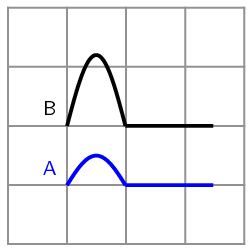Home Practice
For learners and parents For teachers and schools
Textbooks
Full catalogue
Pricing SupportLog in

We think you are located in United States. Is this correct?

# 7.2 Pulses: amplitude and length

## Observation of pulses

Take a heavy rope. Have two people hold the rope stretched out horizontally. Flick the rope at one end only once.

What happens to the disturbance that you created in the rope? Does it stay at the place where it was created or does it move down the length of the rope?

In the activity, we created a pulse. A pulse is a single disturbance that moves through a medium. In a transverse pulse the displacement of the medium is perpendicular to the direction of motion of the pulse. Figure 7.1 shows an example of a transverse pulse. In the activity, the rope or spring was held horizontally and the pulse moved the rope up and down. This was an example of a transverse pulse.

Pulse

A pulse is a single disturbance that moves through a medium.

Transverse Pulse

A pulse where all of the particles disturbed by the pulse move perpendicular (at a right angle) to the direction in which the pulse is moving.

temp text

#### Pulse length and amplitude

The amplitude of a pulse is a measurement of how far the medium is displaced momentarily from a position of rest. The pulse length is a measurement of how long the pulse is. Both these quantities are shown in Figure 7.1.

Amplitude

The amplitude of a pulse is the maximum disturbance or distance the medium is displaced from its rest (equilibrium) position.

Quantity: Amplitude (A)         Unit name: metre         Unit symbol: m

The position of rest is the position the medium would be in if it were undisturbed. This is also called the equilibrium position. People will often use rest and equilibrium interchangeably.

temp text

## Pulse length and amplitude

The graphs below show the positions of a pulse at different times.

Use your ruler to measure the lengths of $$A$$ and $$p$$. Fill your answers in the table.

 Time $$A$$ $$p$$ $$t = \text{0}\text{ s}$$ $$t = \text{1}\text{ s}$$ $$t = \text{2}\text{ s}$$ $$t = \text{3}\text{ s}$$

What do you notice about the values of $$A$$ and $$p$$?

In the activity, we found that the values for how high the pulse ($$A$$) is and how wide the pulse ($$p$$) is the same at different times. Pulse length and amplitude are two important quantities of a pulse.

#### Pulse speed

Pulse speed

Pulse speed is the distance a pulse travels per unit time.

Quantity: Pulse speed ($$v$$)         Unit name: metre per second         Unit symbol: $$\text{m·s^{-1}}$$

Speed is defined as the distance travelled per unit time (this will be covered in more detail in Motion in One Dimension). If the pulse travels a distance $$D$$ in a time $$t$$, then the pulse speed $$v$$ is:

$v = \frac{D}{t}$

## Worked example 1: Pulse speed

A pulse covers a distance of $$\text{2}$$ $$\text{m}$$ in $$\text{4}$$ $$\text{s}$$ on a heavy rope. Calculate the pulse speed.

### Analyse the question

We are given:

• the distance travelled by the pulse: $$D = \text{2}\text{ m}$$

• the time taken to travel $$\text{2}$$ $$\text{m}$$: $$t = \text{4}\text{ s}$$

We are required to calculate the speed of the pulse.

### Apply the relevant principles

We can use:

$v = \frac{D}{t}$

to calculate the speed of the pulse.

### Do the calculation

\begin{align*} v & = \frac{D}{t} \\ & = \frac{\text{2}\text{ m}}{\text{4}\text{ s}} \\ & = \text{0,5}\text{ m·s$^{-1}$} \end{align*}

### Quote the final result

The pulse speed is $$\text{0,5}$$ $$\text{m·s^{-1}}$$.

temp text

The pulse speed depends on the properties of the medium and not on the amplitude or pulse length of the pulse.

## Pulse speed

Textbook Exercise 7.1

A pulse covers a distance of $$\text{5}$$ $$\text{m}$$ in $$\text{15}$$ $$\text{s}$$. Calculate the speed of the pulse.

Solution not yet available

A pulse has a speed of $$\text{5}$$ $$\text{cm·s^{-1}}$$. How far does it travel in $$\text{2,5}$$ $$\text{s}$$?

Solution not yet available

A pulse has a speed of $$\text{0,5}$$ $$\text{m·s^{-1}}$$. How long does it take to cover a distance of $$\text{25}$$ $$\text{cm}$$?

Solution not yet available

How long will it take a pulse moving at $$\text{0,25}$$ $$\text{m·s^{-1}}$$ to travel a distance of $$\text{20}$$ $$\text{m}$$?

Solution not yet available

The diagram shows two pulses in the same medium. Which has the higher speed? Explain your answer.Solution not yet available